Electrics Index

Electric Circuit formula

Introduction..... Current Direction..... Ohms law..... Resistances..... Alternating Current..... Kirchoff's Law.....
Superposition Theorem..... Reciprocity Theorem..... Thevenin's Theorem..... Star_Delta Conversions..... Capacitors.....

Introduction

Provided below are notes and various common formula used in designing electrical circuits.

Direction of currents

The terminology when representing the direction of the positive current flow is as follows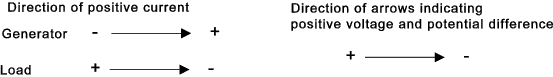Electron drift which is the flow of electrons in an electrical circuit flows from negative to positive .

Electric batteries by convention are identified as shown in the figure below..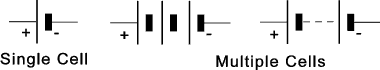A simple dc circuit illustrates the various relationships and directions is shown below..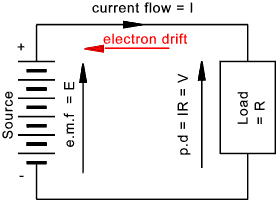Ohms law

 V = Voltage (Volts) I = Current (Amperes) R = Resistance (Ohms) W = Power (Watts) V = I.R = Sqrt (W . R) = W / I I = V / R = Sqrt( W / R) = W / V R = V / I = W / I2 = V2 / W W = VI = V2 / R = I2 . R General Form of Ohms law R = ρ . L / A L = Length (metres), A = Area (metre2 ), ρ = resistivity (Ω /metre)

 Resistors in Series R_total = R 1 + R 2 + R 3... Voltage across Resistors in Series V total = V R1 + V R2 + V R3.. Resistors in Parallel R total = 1/ (1/R 1 + 1/R 2 +1/R 3...) The Voltage across Resistors in Parallel is the Same = V

Alternating Current Supplies

 Alternating Voltage v   =   V max. sin ω. t   =   V max .sin(2.π.f.t) v = instantaneous voltage V_max = maximum voltage, t = time (seconds) from from point of zero rising voltage in sinusoidal variation., ω = angular velocity rads/sec, f = frequency (cycles /sec) The average value of a sinusoidal alternating quantity is calculated at 0.637x maximum value The Root Mean Square(RMS) value of a sinusoidal alternating quantity is calculated at 0.707 x maximum value. The form factor of a wave is the RMS value / average Value = (for a sinusoidal wave) 1.1 The peak (crest) factor is the peak value / RMS value = 1.414

Kirchoff's Laws

 Kirchoffs First law. The total current flowing towards a junction is equal to the total current flowing away from that junction.   i.e the algebraic sum of the currents flowing towards a junction is zero Kirchoff's Second Law In a closed circuit the algebraic sum of the products of the current and the resistance of each part of the circuit is equal to the resultant e.m.f in the circuit. As an example of Kirchoff's second law it is required to find the currents in each branch in the circuit shown below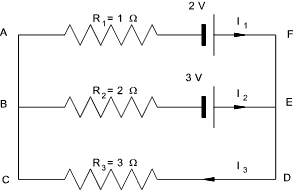First it can be seen that I 3 = I 1 + I 2.......a) In AFEB 2V - 3V = (I 1. 1 Ω) - I 2.2Ω)    Therefore .... -1 A =    I 1 - 2.I 2.......(b) IN BEDC 3V = (I 2.2 Ω) + (I 3.3 Ω )   Therefore.... 3A = 2.I 2 + 3 .I 3 ...... (c) Substituting for I 3 in c) .. 2.I 2 + 3.I 1 + 3.I 2 = 3A    Therefore   3.I 1 + 5.I 2 = 3A .....d) - 3 times b).... -3.I 1 + 6.I 2 = 3A.. and adding the last two equations 11.I 2 = 6A. and therefore I 2 = (6/11) A....     (I 1 = 2.I 2 - 1) ...    so   I 1 =(1/11) A     and    I 3 = I 1 +I 1     so     I 3 = (7/11) A

Superposition Theorem

 Superposition Theorem. This theorem states that in any network containing more than one source , the current in, or the p.d across, any branch can be found by considering each source separately and adding their effects: The omitted sources (of e.m.f) being replaced by resistances equivalent to their internal resistances.

Reciprocity Theorem

 Reciprocity Theorem. This theorem states that if a current is produced at point a in a network by a source acting at point b then the the same current would be produced at b by the same value source acting at point a

Thevenin's Theorem

 Thevenin's Theorem. This theorem is used to determine the voltage / current flow across / through two points say A and B in a complicated circuit which includes and number of sources and loads.    The theorem states The current through a resistance R connected across any two points A and B of an active network ( a network containing one or more sources of e.m.f. ) is obtained by dividing the p.d. between A and B with R disconnected , by ( R + r ) where r is the resistance of the network measure between A and B with R disconnected and the sources of e.m.f replaced by their internal resistance. Consider a general network containing voltage and current sources.  The network can be replaced by a single source of e.m.f (E) with and internal resistance r.   E being the e.m.f. across A- B with the load disconnected and r being the total resistance of the circuit measured across A-B with the load disconnected and the e.m.f. sources replaced by their internal resistances. As an example of this theorem it is necessary to find the p.d across A-B in the figure below.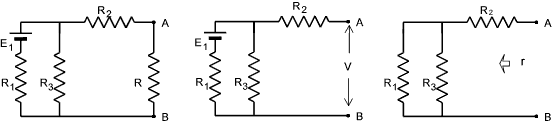First remove the resistance R and calculate V.  As there is no current flow there is no p.d. across R 2 The current through R 3 is E 1 / (R 1 + R 3).. The p.d. across R 3 = ....V = is E 1. R 3 / ( R 1 + R 3) The resistance of the network =   r = R 2 + (R 1 .R 3) / (R 1 + R 3) Thevenin's theorem states that the circuit above can be replaced by the simple circuit as shown below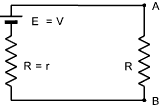The current flow I = V / (r +R)..The pd. across R = V.R/ (r+R)

Start Delta Conversions

 The star delta conversion formula are useful if loads are connected in a star or delta arrangement and it is more convenient to convert to the other arrangement which may be more simpler to resolve for a particular problem....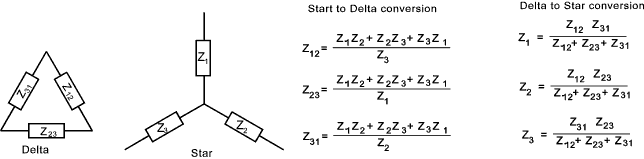The relationships are properties of the load impedances and are independent of the voltages and does not imply three phase working....

Capacitors

 Q = V . C   therefore    I = C dV/dt Q = Charge (Coulomb) V = Potential Difference (Volts) C = Capacitance (Farad) V = V / X c X c = Capacitive Reactance (Ω ) X c = 1 / (2 π f C ) f = frequency (Herz)

 Capacitors in Parallel C total. V = Q total = Q 1+Q 2+Q 3... C total. V = V.C 1+V.C 2+V.C 3... C total = C 1+C 2+C 3... Capacitors in Series 1/C total = 1/C 1 + 1/C 2 + 1/C 3... C total = 1/ (1/C 1 + 1/C 2 + 1/C 3...)

Inductances

 V = L.dI/dt L = Inductance (Henry) I = V/ X L X L = Inductive Reactance (Ω ) X L =2 π f L f = frequency (Herz)